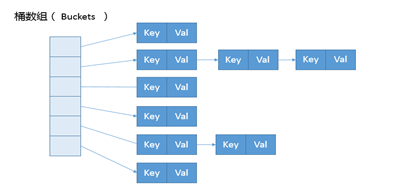# HashMap 源码总结Geek_571bdf

1. HashMap 结构、理解散列表；

2. 理解散列函数；

3. HashMap 是如何确定下标的；

4. equals 和 hashCode 规范

6. 树化与反树化

7. 谈谈 HashMap 在并发环境可能出现无限循环占用 CPU、size 不准确等诡异的问题。

// 阅读时重点阅读 putVal()，初始化、扩容、树化都与它有关。

1. HashMap 的整体结构：Node<K,V>[] table;1. 容量与负载因子。

• 散列函数计算得到的散列值是一个非负整数；

如果 key1 = key2，那 hash(key1) == hash(key2)；

如果 key1 ≠ key2，那 hash(key1) ≠ hash(key2)。

size < thresholdthreshold = loadFactor * capacityif (++size > threshold)    resize(); // 2倍

3. HashMap 源码总结。

Node<K,V>[] table

HashMap 构造器并不初始化数组，对数组的初始化在 resize()中。putàputValàresize()。即，resize()兼顾扩容和对数组的初始化。

2. 总结。

②扩容是扩大 2 倍。// newCap = oldCap << 1、newThr = oldThr << 1; // double threshold

③数组搬迁由于 数组容量 变了，因此需要重新计算下标。然后再将旧数组中的元素搬迁到新数组。

④HashMap 解决散列冲突的方法是：链表+红黑树。当某个桶的链表长度超过 TREEIFY_THRESHOLD(默认 8)，且散列表容量超过 MIN_TREEIFY_CAPACITY（64），则会进行树化。当红黑树节点好于 8 个时，又会反树化成链表。

⑤HashMap 为什么要树化？

⑥树化相关代码：

static final int TREEIFY_THRESHOLD = 8;static final int UNTREEIFY_THRESHOLD = 6;final V putVal(int hash, K key, V value, boolean onlyIfAbsent,               boolean evict) {p = tab[i = (n - 1) & hash];// p为第一个元素//……Node<K,V> e; K k;for (int binCount = 0; ; ++binCount) {	  if ((e = p.next) == null) {	    p.next = newNode(hash, key, value, null);	    if (binCount >= TREEIFY_THRESHOLD - 1) // -1 for 1st	        treeifyBin(tab, hash);	    break;	  }	  p = e;   }}final void treeifyBin(Node<K,V>[] tab, int hash) {    int n, index; Node<K,V> e;    if (tab == null || (n = tab.length) < MIN_TREEIFY_CAPACITY)        resize();    else if ((e = tab[index = (n - 1) & hash]) != null) {        //树化改造逻辑    }}static final int MIN_TREEIFY_CAPACITY = 64;

• 如果容量小于 MIN_TREEIFY_CAPACITY，只会进行简单的扩容。

• 如果容量大于 MIN_TREEIFY_CAPACITY ，则会进行树化改造。

3. HashMap 如何确定元素的下标。

// putVal(hash(key), key, value, false, true);final V putVal(int hash, K key, V value, boolean onlyIfAbsent,               boolean evict) {Node<K,V>[] tab; Node<K,V> p; int n, i;n = (tab = resize()).length; if ((p = tab[i = (n - 1) & hash]) == null)    	tab[i] = newNode(hash, key, value, null);static final int hash(Object key) {    int h;    return (key == null) ? 0 : (h = key.hashCode()) ^ (h >>> 16);}

1. 解读 h^(h>>>16);

h>>>16，将 h 无符号右移 16 位。h 是 int 类型，有 32 位，因此相当于是将高位移到低位。

^ 异或：a b 相同，异或为 1；a b 不同，异或为 0

h^(h>>>16);

h 高位……h 高位^h 低位

2. 解读(n-1) & hash

1AND1=1; 1AND0=0; 0AND0=1

a & b 所得到的值，小于等于 min(a,b)，比如，0011&1101=0001，不会超过 0011

3. 为什么要将 h 的高位移动到低位，并对其进行异或操作呢？## 评论